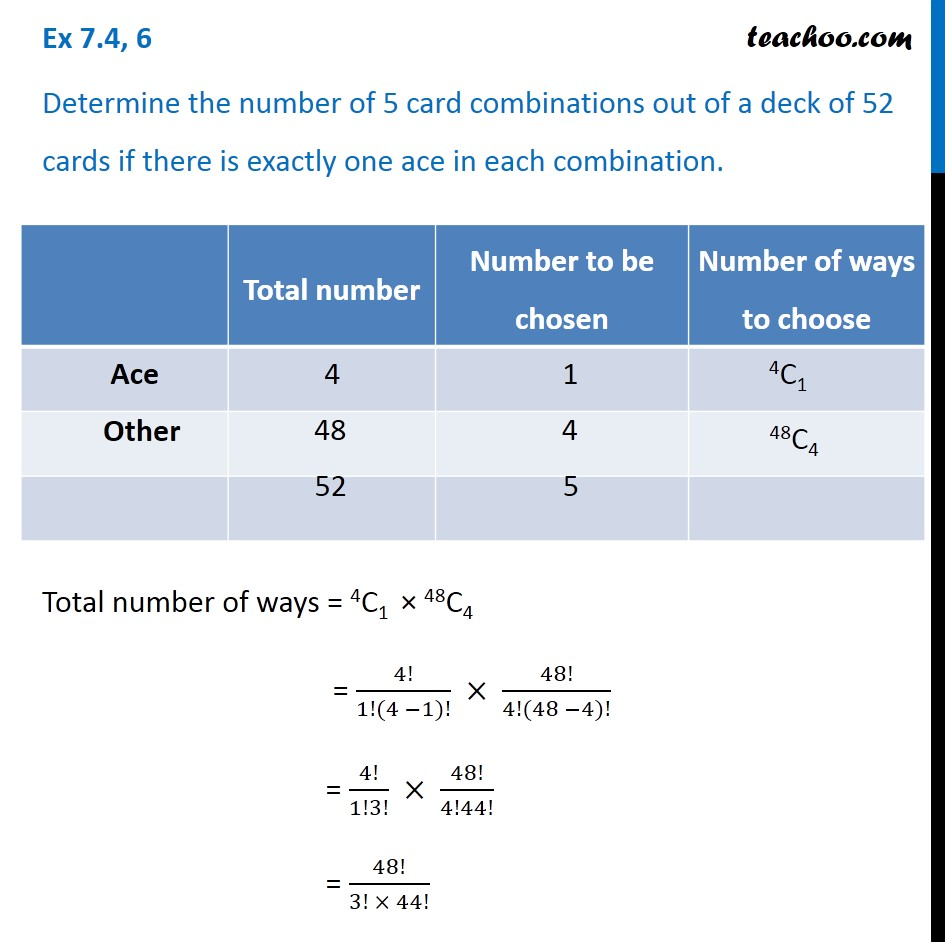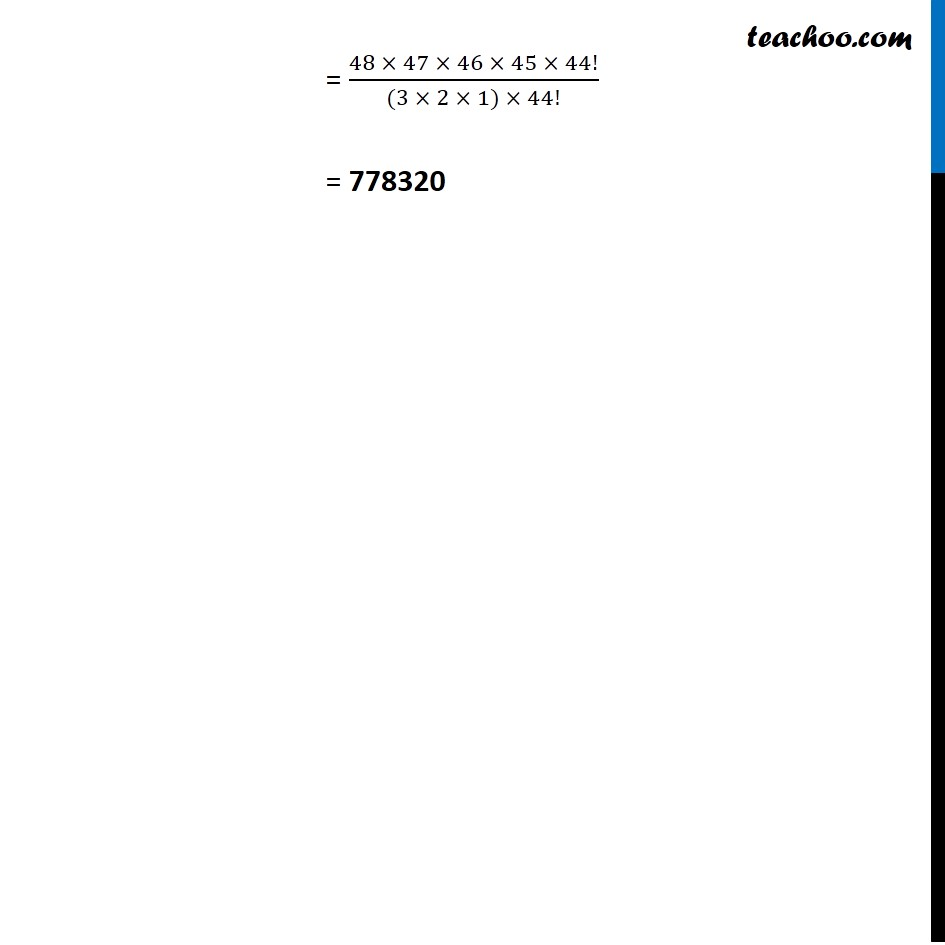1. Chapter 7 Class 11 Permutations and Combinations (Term 2)
2. Serial order wise
3. Ex 7.4

Transcript

Ex 7.4, 6 Determine the number of 5 card combinations out of a deck of 52 cards if there is exactly one ace in each combination. There are total 4 Ace Cards out of 52 We have to select one ace from 4 ace Total number of ways = 4C1 × 48C4 = 4!/(1!(4 −1)!) × 48!/(4!(48 −4)!) = 4!/1!3! × 48!/4!44! = 48!/(3! × 44!) = (48 × 47 × 46 × 45 × 44!)/((3 × 2 × 1) × 44!) = 778320